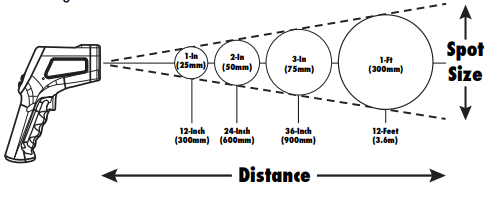### Non Contact Infrared Thermometer

Follow

If the temperature is inaccurate with the non contact infrared thermometer:

Verify size of target is within the field of measurement according to the D:S ratio.

1. The Infrared Thermometer measures average surface temperature on the basis of distance to spot diameter (D:S) ratio.  This ratio is the size of the area being measure by the thermometer as it relates to distance.  The targeted area being measured becomes larger as the distance from the thermometer increases.  The smaller the target the close the thermometer should be to it.Estimate emissivity value of target surface.

1. Emissivity is the measurement of an object's ability to radiate heat.  Emissivity values range from 0 (shiny mirror) to 1.0 (blackbody).  Most organic materials and painted or oxidized surfaces have an emissivity close to .95.  This thermometer is preset (non-adjustable) to an emissivity value of .95.

Note: If you need to measure the temperature of a shiny surface, cover the area with a piece of masking tape. Then, measure the temperature of the taped surface.

Was this article helpful?
0 out of 0 found this helpful